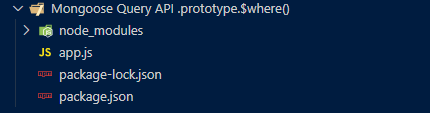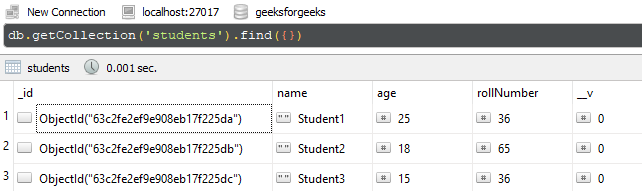GFG App
Open AppBrowser
Continue

# Mongoose Query.prototype.\$where() API

The Mongoose Query API.prototype.\$where() method of the Mongoose API is used on the Query objects. It allows us to put the where condition in the form of JavaScript object or a function in order to pass the expression to the mongodb system. Let us understand the \$where() method using an example.

Syntax:

`query.\$where( js );`

Parameters: This method accepts a single parameter as described below:

• js: It is used to specify the JavaScript expression or function.

Return Value: This method returns query object.

Setting up Node.js Mongoose Module:

Step 1: Create a Node.js application using the following command:

`npm init`

Step 2: After creating the NodeJS application, Install the required module using the following command:

`npm install mongoose`

Project Structure: The project structure will look like this:Database Structure: The database structure will look like this, the following database present in the MongoDB.Example 1: The below example illustrates the basic functionality of the Mongoose Connection \$where() method. We are fetching the document from collection where name is equal to “Student1”.

Filename: app.js

## Javascript

 `// Require mongoose module ` `const mongoose = require(``"mongoose"``); ` ` `  `// Set Up the Database connection ` `const URI = ``"mongodb://localhost:27017/geeksforgeeks"``; ` ` `  `const connectionObject = mongoose.createConnection(URI, { ` `    ``useNewUrlParser: ``true``, ` `    ``useUnifiedTopology: ``true``, ` `}); ` ` `  `const studentSchema = ``new` `mongoose.Schema({ ` `    ``name: { type: String, required: ``true` `}, ` `    ``age: Number, ` `    ``rollNumber: { type: Number, required: ``true` `}, ` `}); ` ` `  `const StudentModel = connectionObject.model(``'Student'``, studentSchema); ` ` `  `const query = StudentModel.find(); ` `query.\$where(``"this.name == 'Student1'"``); ` `query.then(res => { ` `    ``console.log(res); ` `}).``catch``(err => console.log(err));`

Step to run the program: To run the application execute the below command from the root directory of the project:

`node app.js`

Output:

```[
{
_id: new ObjectId("63c2fe2ef9e908eb17f225da"),
name: 'Student1',
age: 25,
rollNumber: 36,
__v: 0
}
]```

Example 2: The below example illustrates the basic functionality of the Mongoose Connection \$where() method. We are fetching the documents from the collection where age is greater than and equal to 18.

Filename: app.js

## Javascript

 `// Require mongoose module ` `const mongoose = require(``"mongoose"``); ` ` `  `// Set Up the Database connection ` `const URI = ``"mongodb://localhost:27017/geeksforgeeks"``; ` ` `  `const connectionObject = mongoose.createConnection(URI, { ` `    ``useNewUrlParser: ``true``, ` `    ``useUnifiedTopology: ``true``, ` `}); ` ` `  `const studentSchema = ``new` `mongoose.Schema({ ` `    ``name: { type: String, required: ``true` `}, ` `    ``age: Number, ` `    ``rollNumber: { type: Number, required: ``true` `}, ` `}); ` ` `  `const StudentModel = connectionObject.model(``'Student'``, studentSchema); ` ` `  `const query = StudentModel.find(); ` `query.\$where(``function` `() { ` `    ``return` `this``.age >= 18; ` `}); ` `query.exec((error, result) => { ` `    ``if` `(error) { ` `        ``console.log(``"Error -"``, error); ` `    ``} ``else` `{ ` `        ``console.log(``"Result -"``, result); ` `    ``} ` `})`

Step to run the program: To run the application execute the below command from the root directory of the project:

`node app.js`

Output:

```Result - [
{
_id: new ObjectId("63c2fe2ef9e908eb17f225da"),
name: 'Student1',
age: 25,
rollNumber: 36,
__v: 0
},
{
_id: new ObjectId("63c2fe2ef9e908eb17f225db"),
name: 'Student2',
age: 18,
rollNumber: 65,
__v: 0
}
]```

My Personal Notes arrow_drop_up